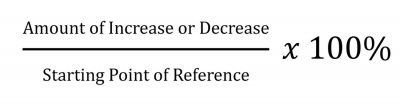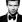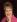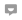# A Simple Explanation on How to Work Percentages

Knoji reviews products and up-and-coming brands we think you'll love. In certain cases, we may receive a commission from brands mentioned in our guides. Learn more.
A Simple Explanation on How to Work Percentages

While percentages are common in everyday life, for most people using percentages correctly is somewhat of a mystery. If you look around you will find their use in many places. You find them advertised at retail stores whenever there is a discount; you see percentages used in newspapers and magazines describing how something has increased or decreased compared to something else. For example: An article will state that gas prices went up 5% over the previous month. In this case the percentage is used to compare the cost of gasoline over two periods of time. Percentages are commonly used by people in every walk of life. The key is how to correctly use them. This short article will give you some simple hints on how to use percentages correctly.

All percentages are basically a number multiplied by 100 with the percentage sign, %, placed to the right of the number: Take for example how 35% and 200% are calculated. The number 2 is equal to 200% and 0.5 is equal to 50%. Likewise a small number like 0.004 is converted to 0.4%.

The math is simple: Take the number you want converted to a percentage and multiply it by 100. For example: 5 turns into 5 x 100% = 500%, 0.35 turns into 0.35 x 100% = 35%, and .004 turns into .004 x 100% = 0.4%.

The reverse is as simple. To convert a percentage to a number you simply divide by 100 and remove the percentage sign from the result. For Example: 500% turns into 500%/100 = 5, 35% turns into 35%/100 = 0.35, and 0.4% turns into 0.4%/100 = 0.004.

The tricky part of dealing with percentages is when you are comparing two groups to each other to determine how one has increased or decreased relative to the other one. Let us consider a simple example: Let us say you start with 100 pennies and someone gives you 25 pennies. Your total is now 125 pennies. If you wanted to compare the increase in the number of pennies as a percentage, how would you do it?

The problem is simple:Let's look at the problem in detail:

Amount of increase or decrease ------------------------------------------------------- Starting point of reference

25 pennies were given --------------------------------------------------------- the starting point of reference is 100 pennies

25/100 x 100% = 25%

Thus, the increase is 25% from what you started. Keep in mind you are dividing the number of pennies you got from what you started with, not the number of pennies that you currently have.

Now let’s look at the same problem from the other direction. If you started with 125 pennies and you gave way 25 pennies, you would have 100 pennies. The question is what percentage of pennies you gave away.

The problem is simple when using the above formula;

Amount of increase or decrease  -------------------------------------------------- Starting point of reference

25 pennies were given--------------------------------------------------------the starting point of reference is 125 pennies

25/125 x 100% = 20%

Take a moment to look at both of these two results. In the first case you increased the amount of pennies by 25%. In the second case you decreased the amount of pennies by 20%. Two are different answers resulting from the same number of 25 pennies changing hands. How can this be?

The answer depends on your starting point of reference. In the first case you only had 100 pennies and added 25 more pennies. In the second case you started with 125 pennies, not 100 pennies, and gave away 25 pennies. The difference is what you started in the beginning of the problem: 100 versus 125 pennies.

That is why percentages are tricky. They take into account the direction of comparison, not just the difference between the two amounts!

The key is always to begin with what you are comparing, NOT what your ending result will be. If you use the formula given above you will get it correct every time.

Lastly, a simple problem for you:

If you start with 10 students and add another 10 students, what is the percentage increase in the number of students added? Likewise if you start with 20 students and lose 10 students, what is the percentage decrease? Try your luck. If you get 100% as an answer for the first question and 50% as an answer for the second question then you have mastered percentages. If not, don't worry, you will get it correct on the second attempt.Aunty Ann
0
Posted on Apr 30, 2011Lorena Williams
0
Posted on Apr 28, 2011Eva Coombes
0
Posted on Apr 27, 2011Lorena Williams
0
Posted on Apr 27, 2011